# Using Linear Inequalities in Real World Scenarios

Author: Sophia Tutorial
##### Description:

Predict a possible solution to a linear inequality.

(more)

Sophia’s self-paced online courses are a great way to save time and money as you earn credits eligible for transfer to many different colleges and universities.*

No credit card required

46 Sophia partners guarantee credit transfer.

299 Institutions have accepted or given pre-approval for credit transfer.

* The American Council on Education's College Credit Recommendation Service (ACE Credit®) has evaluated and recommended college credit for 33 of Sophia’s online courses. Many different colleges and universities consider ACE CREDIT recommendations in determining the applicability to their course and degree programs.

Tutorial
what's covered
1. Linear Inequalities representing a Budget/Cost Restraint
2. The Graph of a Linear Inequality
3. Using the Inequality and Graph to Solve Problems

# 1. Linear Inequalities representing a Budget/Cost Restraint

Many event planners and project managers that put on events have a certain budget they must stick to. This budget represents a maximum amount of dollars that you can spend on the event or project. Suppose that you are an event planner with a certain budget planning a company's promotional event.

One of the company's board members wants as many balloons at the party as possible. You would like to meet the client's desires, but you have already dedicated \$1730 of your budget to other expenses. After researching the cost for balloons, you figure out that each balloon will cost about \$1.30 in all - this includes the purchase of each balloon, and the helium for the tank.

We can represent this scenario with the following inequality: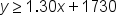On one side of the inequality, we have our budget of y–dollars. On the other side, we have our expression for the cost of the event, \$1.30 per balloon, plus \$1730 in other costs. We can see that x represents the number of balloons. The inequality symbol we use is the "greater than or equal to" sign, because we want our budget to remain greater than our cost (this means we'll have money to spare), but we are also allowed to spend exactly all of the money we have.

# 2. The Graph of a Linear Inequality

To graph the inequality for our situation, we need to analyze the inequality symbol. Inequality signs that include "or equal to" are drawn with a solid line on the graph, to show inclusion of the exact values that the line graphs. If the symbol does not include "or equal to" then we use a dashed line to show that we are not accepted the exact values on the line as solutions to our inequality.

We start by drawing the boundary line, which is the equation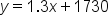: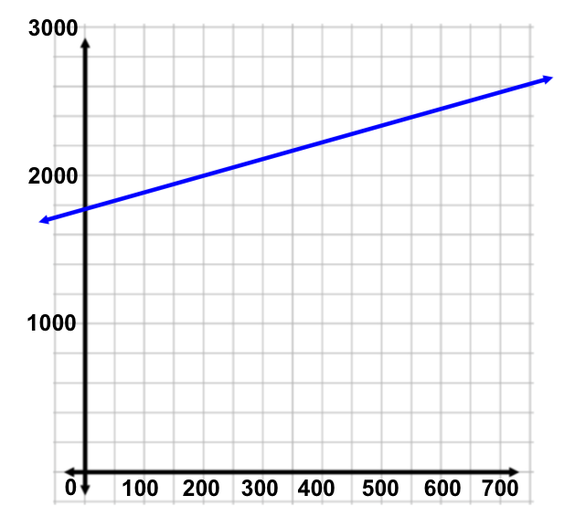Then we need to shade a region of the coordinate plane, to highlight coordinate pairs that satisfy our inequality. We can use a test point to do so. Whenever possible, try choosing the origin (0, 0). This is because it is easy to multiply and add with zero when making calculations. If the test point satisfies the inequality, we shade the portion of the coordinate plan that includes the test point. If not, we shade the other portion of the coordinate plane.Our inequality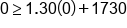Using the origin: x=0, y=0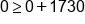Evaluate 1.30(0)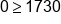False statement

This means we shade the other part of the graph. We can call this region the solution region or feasibility region. Every coordinate point (x, y) represents the maximum number of balloons we can spend, x, given that particular budget, y.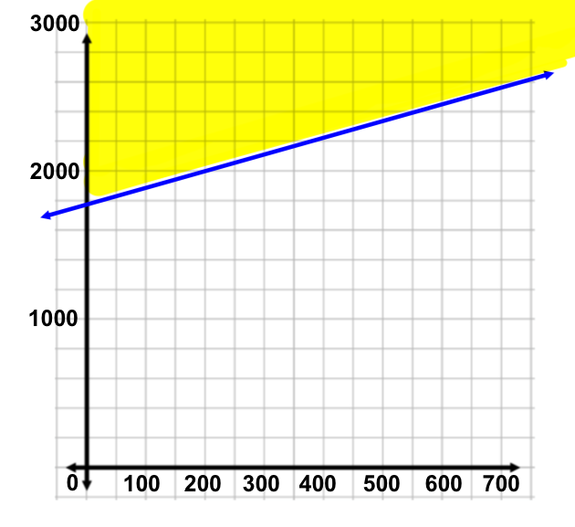hint
In real world scenarios, the inequalities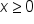and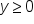are often natural boundaries (we cannot buy negative balloons, and our budget cannot be negative dollars). This is why the negative sides of both x– and y– on the coordinate plane are not part of the solution region)

# 3. Using the Inequality and Graph to Solve Problems

Let's use the graph and the inequality to answer some questions about our scenario.

QUESTION 1

Can we afford to purchase 700 balloons for the event with a budget of \$2500?

To answer this question, we plug 700 in for x, and 2500 in for y. If the statement is true, we can afford 700 balloons with a budget of \$2500.Our inequality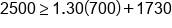x=700, y=2500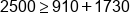Evaluate 1.30(700)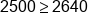False statement

This means we cannot afford 700 balloons with a budget of \$2500.

QUESTION 2

How many balloons can we purchase with a budget of \$2000?

Let's first use the graph to get an estimate. We can add a horizontal line at y = 2000, and find where the line intersections the boundary line. This would represent the maximum number of balloons we can buy with a budget of \$2000.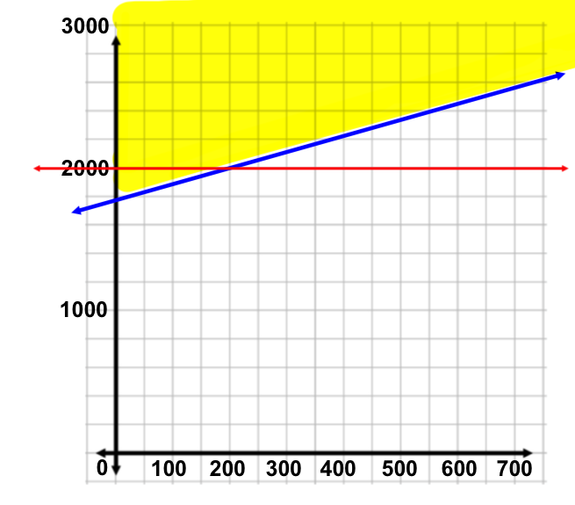According to the graph, we estimate that we can buy no more than 200 balloons with our budget.

We can get an exact answer using the inequality and solving algebraically:Our inequality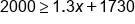y=2000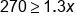Subtract 1730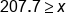Divide by 1.3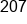Our solution

hint
Because x represents number of balloons, it doesn't make sense that our answer remain as a decimal. Therefore, we have to express our answer as a whole number. But in real world scenarios, standard rounding rules might not make sense. In this example, we had to round 207.7 down to 207, because if we rounded to 208, we would be accepting 208 as an answer to the inequality, which would be false. 208 balloons would put the event cost at \$2000.40, which is slightly over our budget.

summary
A linear inequality can be used for scenarios limited by certain constraints, such as linear inequalities representing a budget/cost restraint. With the graph of a linear inequality, recall that the inequality symbols indicate if we use a dashed or solid line and if we shade above or below the line. We can use the inequality and graph to solve problems that model the scenario and observe the solution region.

Rating